# “Analytic” Math: A Variant to the Pure-Applied Paradigm

In Math by Alon K.

As an applied mathematician – and in particular, working now in the field known as “Data Science” – I have developed a unique work routine through the years to the research and development process. It is unique in the sense that I do not often see it used in practice but I often see that this process leads to results. To contrast, I also see many in the field simply try to guess their way to success and often fail – although some strike it big, once, but only that one time. And therein lies the difference, consistently delivering results!

I owe most of this success to my strict emphasis on the importance of understanding the “why” of mathematics, rather than just taking some tools and applying them at face value. Often, such boilerplate philosophical treatment of science falls in the realm of pure mathematics, and if not for the rigours of pure math, I can candidly say that fields like physics, chemistry, etc… (the “exact” sciences) would be no better off today than, say, sociology, nutrition, etc… where the rules of math are bent so that the results can come out one way or another.

In this article I will go over one experience during my undergrad years that shows just how powerful it is to have a strong grasp of pure math, and how pure math isn’t just some philosophical voodoo mental masturbation, but can lead to real world application. As such, I find it better calling this viewpoint neither “pure” nor “applied” math, but something in between – perhaps “analytic” math. And so on with the story…

When I attended university, I was rather undecided about my major. I did, however, know that I liked science (particularly physics) and that I liked math. So I was an undeclared major for my first semester – completely normal at an American university.

Among the many courses I had during that first semester, one class that I took was called Real Analysis, and another was Physics 2 (involving the study of electrodynamics and optics) – I skipped Physics 1 because I negotiated with the department head that I know all the material taught in both Physics 1 & 2, so he agreed that I could simply skip Physics 1 if I passed the final exam before the semester began, and I did.

In Real Analysis I studied how to construct the proofs underlying single-valued Riemannian calculus – involving such things as continuity, sequences, series, differentiation, integration, and a tiny bit about the Fourier transform. While in Physics 2 I was learning about how light and charged matter behaves over time, using the very tools I learned so deeply about in Real Analysis. So where in Real Analysis I might ask “why does this even matter?”, in Physics I could ask “why is it so?”. If not for taking both classes simultaneously, I might actually have taken such “why” questions to the professors of those classes.

Nevertheless, one day I did indeed have to do this in my Physics class. We looked at Maxwell’s version of Ampere’s Law, and I had a hard time accepting Maxwell’s version of things. I took my concerns to the professor and asked him “why should I believe Maxwell, when Ampere gave a convincing argument as to how current flows”, and “how can both of them be correct, because they lead to different results.”

Before going further, let’s have a crash course on Ampere’s Law.

In the 1820’s, André-Marie Ampère (Ampere) studied the relationship between electricity and magnetism, following Hans Christian Ørsted’s demonstration that a magnetic needle is deflected by an electric current running in an adjacent wire. Ampere discovered in his experiments that two wires at a distance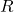with current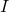are attracted/repelled to one another, like magnets, with the following relationship: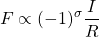Where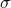is 0 or 1 based on whether the current is in the same or opposite directions, respectively, and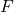is the magnetic force of attraction (with a negative force representing repulsion). Further experiments, showed that this force is none other than magnetism, because it caused the same kind of behaviour in metals as did magnets.

Now, applying some multi-variate calculus, and adding a constant of proportionality, one can arrive at the general relationship known today as Ampere’s Law: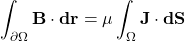Where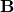is the magnetic field,is an oriented surface in space with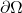as its oriented closed boundary,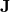is the electric current density field(per unit area), and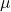is the proportionality constant known as the permeability of space. In words, Ampere’s Law states that the magnetic field along a closed path generates, or is generated by, an electric current passing through the surface formed by that path. But we are already getting a bit ahead of ourselves, as this multivariate formulation only came about in 1855 when James Clerk Maxwell published it.

Maxwell’s main contributions to the field was to model the electromagnetic phenomena as a mechanical system of fluid flow. He took the various laws relating to electricity and magnetism, and put them together as a set of four equations – the Maxwell equations. But he noticed that Ampere’s Law did not fit this framework.

Firstly, if we rewrite Ampere’s Law as a differential equation, we have: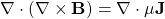And because the divergence of the curl must equal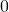, then so must the above equation. So we actually have:But this is a problem, because as charge leaves a point – forming a current – then the charge density at that point should decrease: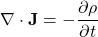And this is also confirmed experimentally.

This actually happens in circuits with a capacitor, where charge enters/exits on the wired side of the capacitance plate, but does not exit/enter on the gap side of the plate. So charge density builds up or depletes over time, and the divergence of the curl of the magnetic field cannot be zero. So in 1861, Maxwell published an updated equation of Ampere’s Law that accommodates for currents that change over time as follows: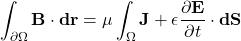Where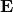is the electric field. So let’s get back to our main topic – analytic math.

If you paid close attention to the previous section, you will notice that what invalidated Ampere’s Law was not an experiment, but rather that it was inconsistent with a fluid mechanic (or vector calculus) model of electromagnetic phenomena when incorporating other laws – in this case, the conservation of electric charge. Math caught the incompleteness!

But this is not how it was taught to me in class, for several reasons. Firstly, physics is an observation driven science, and as such, observations should prompt a need to change Ampere’s Law. Secondly, undergraduate physics courses generally don’t require knowing vector calculus, or where they do, the problems are posed in such a way that students can rely on single-valued calculus almost entirely. And Finally, even if you do take a vector calculus approach that you learned in your undergraduate classes, there is a good chance that in covering divergence, Green’s, and Stoke’s theorems, you did not receive the sufficient emphasis on the underlying concepts driving these theorems – in short, they relate what happens on the boundaries of a region to what happens within that region.

Plainly put, the reasoning for why Ampere’s Law as it existed originally was not good enough seemed rather random because I did not understand vector calculus. I didn’t realize that there really was no need for alarm, all we were doing was accounting for situations that before we could not have known about. Think of what happens when we differentiate a constant. Regardless of the constant, we would always see a 0. However, this time we are aware of the constant, and we call it the electric field. And when it is not constant, it shows up as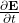.

Just math; no physics. And then it all makes sense!

So when I talk about “analytic math”, I think of this story in my life as a great example. It means that when we apply all these wonderful tools at our disposal to create models of nature, we should not forget how these tools work and where they should fit. We should pay careful attention to the underlying assumptions that we make, because if they are false, then our results will not stand.

So in conclusion, don’t look at “pure” math as useless in applied fields. It’s simply not true. For example, I see a bigger demand for someone that understands what is a Fourier transform and how to correctly apply it to new streams of data, and lower demand for someone that memorized tables of Fourier transforms. I see a bigger demand for people that understand what are measurable sets, pre-images and homeomorphisms, and how to use them to design stable neural networks, than someone who read about every fad network design and has no idea when and why something should work and how to actually make it work in the real world. And finally – last example, I promise – I see a huge demand for people who understand what is a Fisher matrix and how to use it to improve a probabilistic model that only has 10 parameters, rather than someone who takes a 5,000,000 parameter LSTM, gets some results, and makes up some nonsensical reason as to why this approach is state-of-the-art.

But at the same time, math for math’s sake is not that much in demand, either. The real demand is in knowing how to exactly pick out the correct mathematical technique or framework to deliver solutions that already exist to fields that need them. “Pick out the correct mathematical technique or framework” – that is “analytic” math.